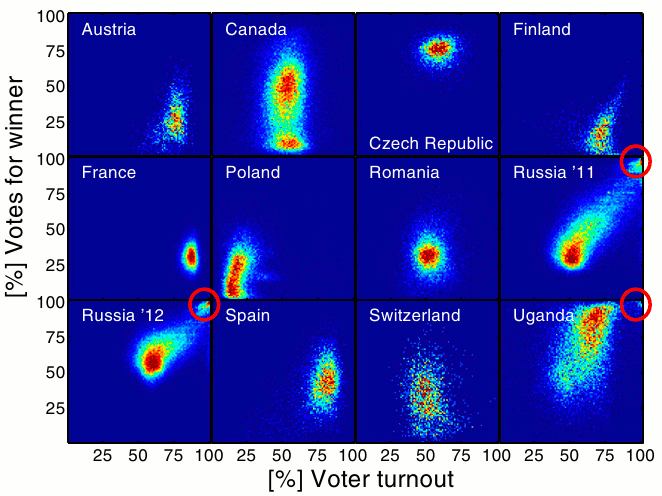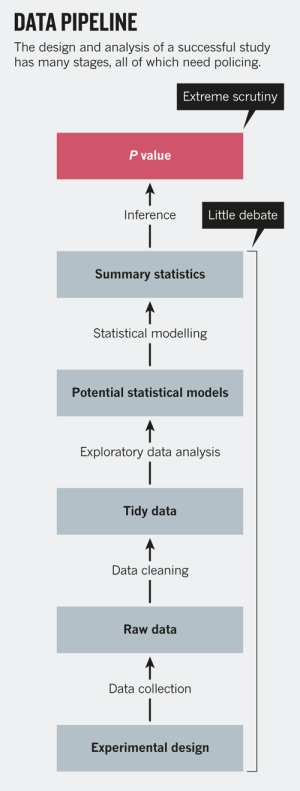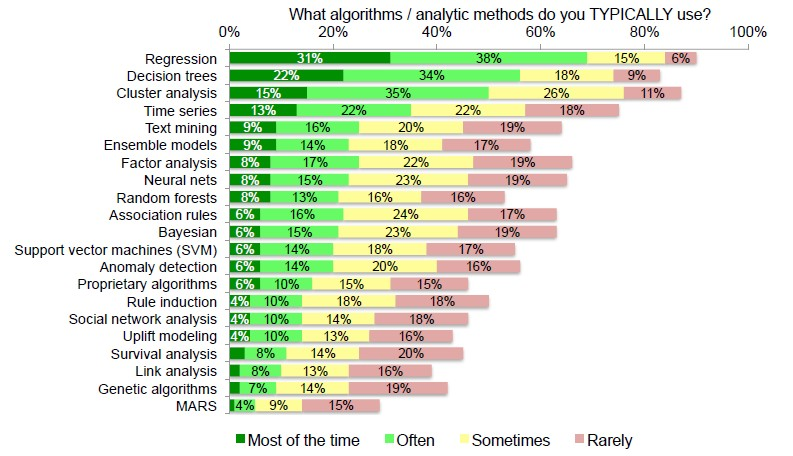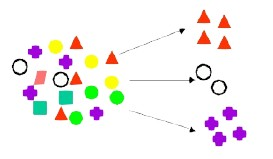# Data Mining - (Classifier|Classification Function)

A classifier is a Supervised function (machine learning tool) where the learned (target) attribute is categorical (“nominal”) in order to classify.

It is used after the learning process to classify new records (data) by giving them the best target attribute (prediction).

Rows are classified into buckets. For instance, if data has feature x, it goes into bucket one; if not, it goes into bucket two.

The target attribute can be one of k class membership.

To summarize the results of the classifier, a Confusion matrix may be used.

## Type

In classification, there is two kind of problem:

• binary
• multi-class

### One

One class classification: Data Mining - (Anomaly|outlier) Detection

### Binary

A two class problem (binary problem) has possibly only two outcomes:

• “yes or no”
• “success” or “failure”

### Multi-class

A multi-class problem has more than two possible outcomes.

## Example

Example Prediction Illustrate the Model
Filter Spam Yes or No Binary Classification
Purchasing Product X Yes or No Binary Classification
Defaulting on a loan Yes or No Binary Classification
Failing in the manufacturing process Yes or No Binary Classification
Producing revenue Low, Medium, High Multi-class Classification
Differing from known cases Yes or No One-class Classification

## Mathematical Notation

The classification task is to build a function that takes as input the feature vector X and predicts its value for the outcome Y i.e.

$C(X) \in C$ where:

• X is a feature vector
• Y is a qualitative response taking values in the set C

C of X gives you values in the set C.

## Probabilities

Often we are more interested in estimating the probabilities (confidence) that X belongs to each category in C.

For example, it is more valuable to have an estimate of the probability that an insurance claim is fraudulent, than a classification fraudulent or not.

You can imagine, in the one situation, you might have a probability of 0.9 the claim is fraudulent. And in another case, it might be 0.98. Now in both cases, those might both be above the threshold of raising the flag that this is a fraudulent insurance claim. But if you're going to look into the claim, and you're going to spend some hours investigating, you'll probably go for the 0.98 first before the 0.9. So estimating the probabilities is also key.

## Data structure / Model

Most of the algorithms are based on this data structure (knowledge representation):

## Logistic Regression versus LDA

• Logistic regression uses the conditional likelihood based on Pr(Y|X) (known as discriminative learning).
• LDA uses the full likelihood based on Pr(X|Y) (known as generative learning).
• Despite these differences, in practice the results are often very similar.

## Summary

• Logistic regression is very popular for classification, especially when K = 2.
• LDA is useful when n is small, or the classes are well separated, and Gaussian assumptions are reasonable. Also when K > 2.
• Naive Bayes is useful when p is very large

## Others

MaxEnt and SVM uses another mathematical models and feature weight selection then Naive Bayes. The feature are more weighed.

## Example

Given demographic data about a set of customers, predict customer response to an affinity card program

## Documentation / Reference

Discover More(Data|Text) Mining - Word-sense disambiguation (WSD)

Word-sense disambiguation (WSD) is an open problem of natural language processing, which governs the process of identifying which sense of a word (i.e. meaning) is used in a sentence, when the word has...(Machine|Statistical) Learning - (Target|Learned|Outcome|Dependent|Response) (Attribute|Variable) (Y|DV)

An (outcome|dependent) variable is ameasure that we want to predict. : the original score collected : the predicted score (or estimator) from the equation. The hat means “estimated” from the...Data Mining - (Anomaly|outlier) Detection

The goal of anomaly detection is to identify unusual or suspicious cases based on deviation from the norm within data that is seemingly homogeneous. Anomaly detection is an important tool: in data...Data Mining - (Class|Category|Label) Target

A class is the category for a classifier which is given by the target. The number of class to be predicted define the classification problem. A class is also known as a label. Labeled...Data Mining - (Discriminative|conditional) models

Discriminative models, also called conditional models, are a class of models used in machine learning for modeling the dependence of an unobserved variable y on an observed variable x. Discriminative...Data Mining - (Function|Model)

The model is the function, equation, algorithm that predicts an outcome value from one of several predictors. During the training process, the models are build. A model uses a logic and one of several...Data Mining - (Life cycle|Project|Data Pipeline)

Data mining is an experimental science. Data mining reveals correlation, not causation. With good data, you will make good algorithm. The most preferable solution is then to work on good features....Data Mining - (Prediction|Guess)

Something predictable is showing a pattern and is therefore not truly random. entropytrue randomness Many forms of data mining model are predictive. For example, a model might predict income based on...Data Mining - Algorithms

An is a mathematical procedure for solving a specific kind of problem. For some data mining functions, you can choose among several algorithms. Algorithm Function Type Description Decision...Data Mining - Clustering (Function|Model)

To identify natural groupings in the data. Useful for exploring data and finding natural groupings within the data. Members of a cluster are more like each other than they are like members of a different...